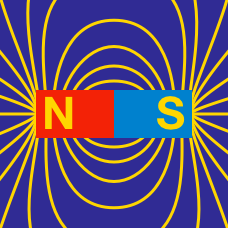Electricity and Magnetism

# Ampere's law (qualitative)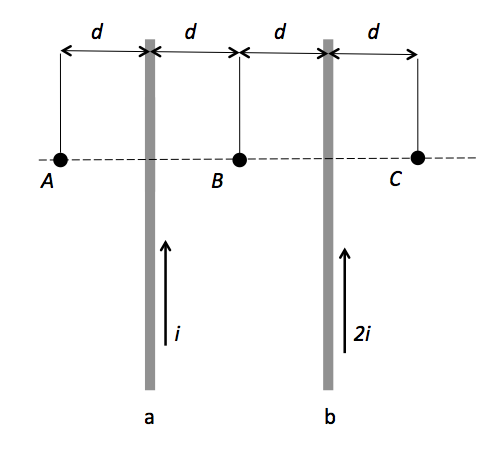A current of $i$ flows through a straight wire $a,$ and a current of $2i$ flows through another straight wire $b$ which is $2d$ away from $a ,$ as shown in the above figure. Which of the following is an appropriate comparison of the magnitudes of the magnetic field strengths at the three points $A, B$ and $C?$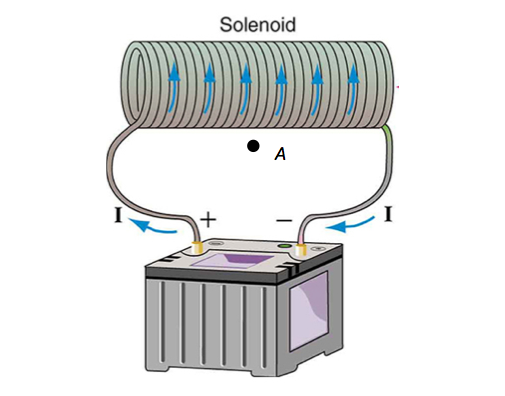A solenoid has current flow $I ,$ as shown in the above figure. What is the direction of the magnetic field at point $A?$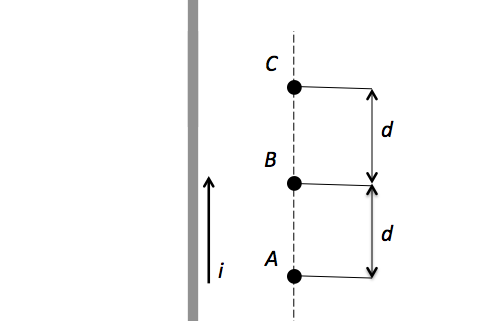Current $i$ flows through a straight wire, as shown in the above figure. Which of the following is an appropriate comparison of the magnitudes of the magnetic field strengths?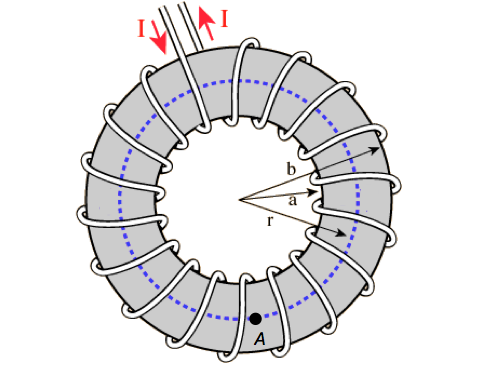A toroid has current flow $I,$ as shown in the above figure. What is the direction of the magnetic field at point $A?$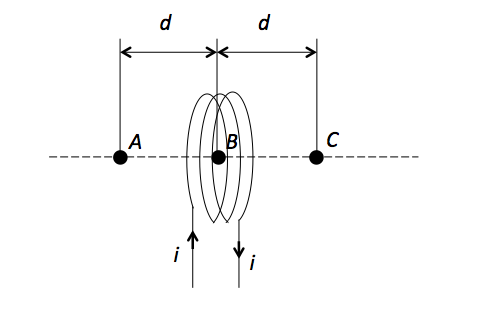A solenoid has current flow $i ,$ as shown in the above figure. Which of the following is an appropriate comparison of the magnitudes of the magnetic field strengths at the three points $A, B$ and $C?$

×